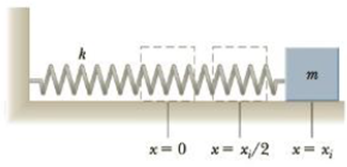Chapter 13, Problem 21P

Chapter
Section
Textbook Problem

A horizontal spring attached to a wall has a force constant of k = 8.50 × 102 N/m. A block of mass m = 1.00 kg is attached to the spring and rests on a frictionless, horizontal surface as in Figure P13.21. (a) The block is pulled to a position xi = 6.00 cm from equilibrium and released. Find the potential energy stored in the spring when the block is 6.00 cm from equilibrium. (b) Find the speed of the block as it passes through the equilibrium position. (c) What is the speed of the block when it is at a position xi/2 = 3.00 cm?Figure P13.21

a)

To determine
The potential energy stored in the spring when the block is 6.00cm from the equilibrium position.

Explanation

Given info: The force constant of the spring is 8.50×102Nm-1 . The mass of the block is 1.00kg . The block is pulled xi=6.00cm from the equilibrium position and released.

Explanation:

The elastic potential energy stored in a system in simple harmonic motion is given by,

PE=12kx2

Here,

PE is the potential energy

k is the force constant of the spring

x is the displacement from the equilibrium

Substituting 8.50×102Nm-1 for k , 6.00cm for x to find the potential energy is,

PE=12(8

b)

To determine
The speed of the block when it passes through the equilibrium position.

c)

To determine
The speed of the block when x=xi2 .

Still sussing out bartleby?

Check out a sample textbook solution.

See a sample solution

The Solution to Your Study Problems

Bartleby provides explanations to thousands of textbook problems written by our experts, many with advanced degrees!

Get Started

In this nation, stringent controls make it difficult to obtain a bogus nutrition credential. T F

Nutrition: Concepts and Controversies - Standalone book (MindTap Course List)

What is the name of the element in Group 4A and Period 5?

General Chemistry - Standalone book (MindTap Course List)

Why is there a mass-luminosity relation?

Horizons: Exploring the Universe (MindTap Course List)

What is the structure and function of the cytoskeleton?

Biology: The Dynamic Science (MindTap Course List)